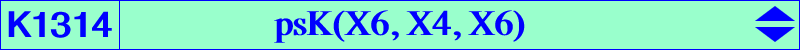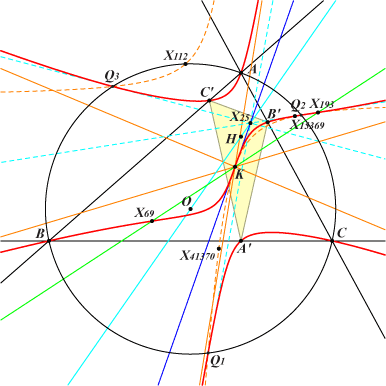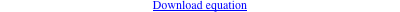too complicated to be written here. Click on the link to download a text file.X(6), X(69), X(193), X(15369) tangential of X(69) = reflection of X(15369) in X(6) = H-Ceva conjugate of X(8770) reflections of A, B, C in X(6) vertices of the orthic triangle and their reflections in X(6) Geometric properties :K1314 is an example of central psK with center X(6). See K1313 for other related properties. The inflexional tangent at X(6) passes through X(25), X(51), X(154), etc. K1314 meets the circumcircle (O) at A, B, C (with tangents passing through O) and Q1, Q2, Q3 (with tangents passing through X25). Q1, Q2, Q3 lie on a hyperbola passing through X(6), X(112), X(193), X(41370), tangent at X(6) to the inflexional tangent above. K1314 meets the line at infinity at the same points as a family of pKs with pole Ω on K009, isopivot Q on K260 and pivot P on K1315, a nodal cubic with node X(69) passing through X(66), X(69), X(193), X(253), X(317). Q is the X(184)-crossconjugate of Ω and, obviously, P is the barycentric quotient Ω ÷ Q, also ta(X184 ÷ Q). Examples : pK(X3, X69), pK(X4, X317), pK(X32, X193), pK(X1147, X40697), pK(X14376, X66), pK(X14379, X253). Under both isogonal conjugation and H-Ceva conjugation, K1314 is transformed into psK(X6, X4, X2). Hence the product of these conjugations in every order keeps K1314 unchanged. K1314 is the image of K830 under SS{a → a^2}.